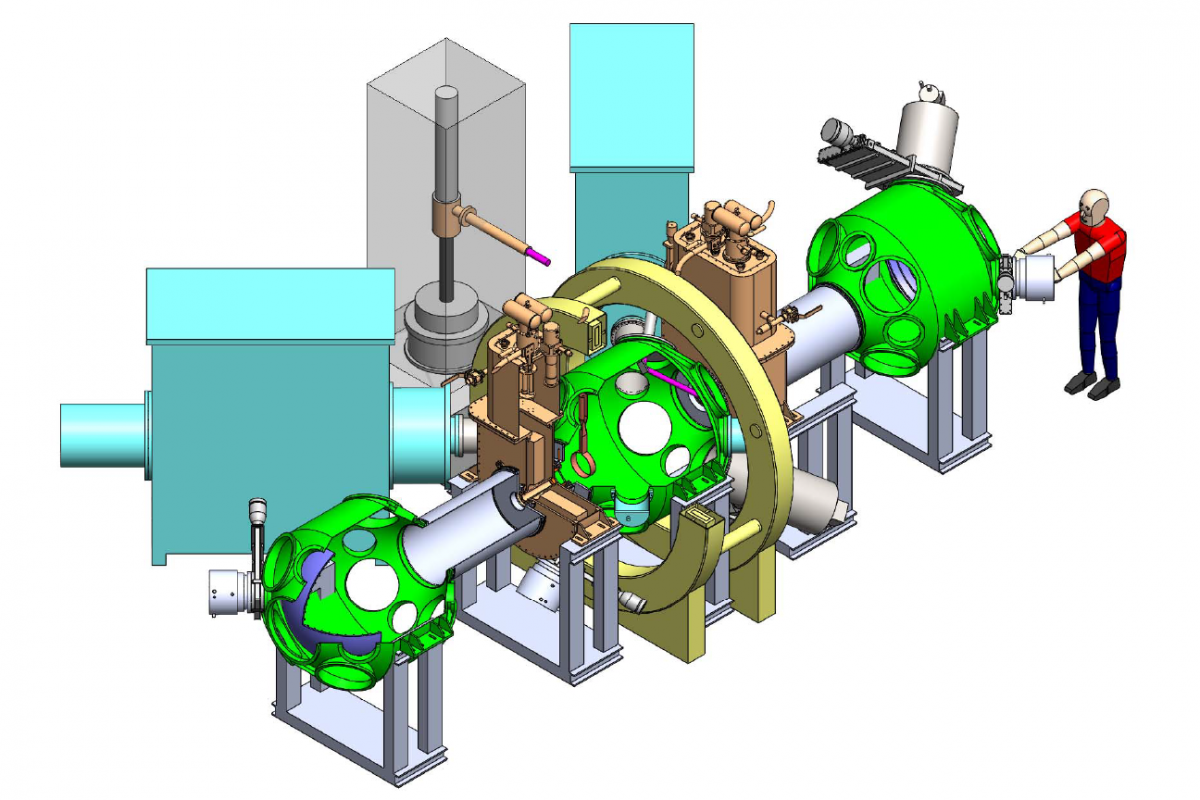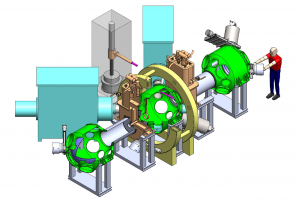# New study expands types of physics, engineering problems that can be solved by quantum computersA well-known quantum algorithm that is useful in studying and solving problems in quantum physics can be applied to problems in classical physics, according to a new study in the journal Physical Review A from University of Wisconsin–Madison assistant professor of physics Jeff Parker.

Quantum algorithms – a set of calculations that are run on a quantum computer as opposed to a classical computer – used for solving problems in physics have mainly focused on questions in quantum physics. The new applications include a range of problems common to physics and engineering, and expands on the types of questions that can be asked in those fields.

“The reason we like quantum computers is that we think there are quantum algorithms that can solve certain kinds of problems very efficiently in ways that classical computers cannot,” Parker says. “This paper presents a new idea for a type of problem that has not been addressed directly in the literature before, but it can be solved efficiently using these same quantum computer types of algorithms.”

The type of problem Parker was investigating is known as generalized eigenvalue problems, which broadly describe trying to find the fundamental frequencies or modes of a system. Solving them is crucial to understanding common physics and engineering questions, such as the stability of a bridge’s design or, more in line with Parker’s research interests, the stability and efficiency of nuclear fusion reactors.

As the system being studied becomes more and more complex — more components moving throughout three-dimensional space — so does the numerical matrix that describes the problem. A simple eigenvalue problem can be solved with a pencil and paper, but researchers have developed computer algorithms to tackle increasingly complex ones. With the supercomputers available today, more and more difficult physics problems are finding solutions.

“If you want to solve a three-dimensional problem, it can be very complex, with a very complicated geometry,” Parker says. “You can do a lot on today’s supercomputers, but there tends to be a limit. Quantum algorithms may be able to break that limit.”

The specific quantum algorithm that Parker studied in this paper, known as quantum phase estimation, had been previously applied to so-called standard eigenvalue problems. However, no one had shown that they could be applied to the generalized eigenvalue problems that are also common in physics. Generalized eigenvalue problems introduce a second matrix that ups the mathematical complexity.

Parker took the quantum algorithm and extended it to generalized eigenvalue problems. He then looked to see what types of matrices could be used in this problem. If the matrix is sparse ­— meaning, if most of the numerical components that make it up are zero — it means this problem could be solved efficiently on a quantum computer.The study shows that quantum algorithms could be applied to classical physics problems, such as nuclear fusion mirror machines. | Credit: Cary Forest

“What I showed is that there are certain types of generalized eigenvalue problems that do lead to a sparse matrix and therefore could be efficiently solved on a quantum computer,” Parker says. “This type includes the very natural problems that often occur in physics and engineering, so this study provides motivation for applying these quantum algorithms more to generalized eigenvalue problems, because it hasn’t been a big focus so far.”

Parker emphasizes that quantum computers are in their infancy, and these classical physics problems are still best approached through classical computer algorithms.

“This study provides a step in showing that the application of a quantum algorithm to classical physics problems can be useful in the future, and the main advance here is it shows very clearly another type of problem to which quantum algorithms can be applied,” Parker says.

The study was completed in collaboration with Ilon Joseph at Lawrence Livermore National Laboratory. Funding support was provided by the U.S. Department of Energy to Lawrence Livermore National Laboratory under Contract No. DE-AC52-07NA27344 and U.S. DOE Office of Fusion Energy Sciences “Quantum Leap for Fusion Energy Sciences” (FWP SCW1680).